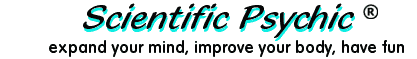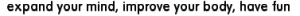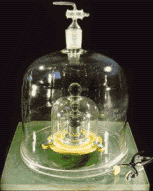Index# The Ship in a Tub Problem - part 1

A toy ship is floating in a cylindrical container 10 centimeters in diameter that is partially filled with water. How much will the level of the water rise if a silver teddy bear weighing 100 grams is loaded on the ship? By the way, silver has a density of 10.49 g/cc.

A 100 gram weight added to the ship will cause the ship to go lower into the water and displace an equal weight of water to continue floating (Archimedes principle of buoyancy). According to the Merk Index, the density of water is 0.997 grams/cubic centimeter at room temperature and, therefore, the volume of water displaced is 100 g / (0.997 g/cm3) = 100.3 cc. The density of the object loaded on the ship is not relevant.

The diameter of the cylindrical container is 10 cm and its volume is calculated by the formula V = πr2h, where r = 5 cm. Solving for h we can calculate the change in water level caused by a volume change V of 100.3 cc.

h = V/(πr2) = 100.3 cm3 / (3.1416 × 25 cm2) = 1.28 cm

The level of the water will go up by 1.28 centimeters (12.8 millimeters).

Archimedes lived between 287 BC and 212 BC and was the most famous ancient Greek mathematician and inventor. He obtained the first approximation of Pi(π) as 3.14 by inscribing and circumscribing regular polygons inside and outside a circle, and computing the perimeter of the polygons. When Archimides was asked to determine the purity of the metal in a gold crown, he got the idea for the principle of buoyancy when his bathtub overflowed as he stepped into the tub. He reportedly was so excited that he ran into the street naked shouting "eureka" (I found it!).

The kilogram was originally defined in the eighteenth century as the mass of one cubic decimeter (one liter) of water at standard pressure and at 3.98° Celsius, which is the temperature at which water has its maximum density. Because of this definition, water has a density close to 1 g/cc. The density of water decreases to 0.917 g/cc when it freezes, which is why ice floats on water (approximately 8% of the ice is above the water line). Improvements in measurement techniques caused the kilogram to be re-defined as the mass of a particular prototype made of a platinum-iridium alloy kept at the International Bureau of Weights and Measures in Sèvres, a suburb south-west of Paris, France.

The meter was originally defined as one ten-millionth of the distance from the geographic north pole to the equator, but it has been re-defined as the length of the path traveled by light in vacuum during a time interval of 1/299,792,458 of a second.The kilogram prototype is kept in a vacuum jar.